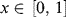Problem 22

Borel and A Different Kind of Normality (1909)

Problem. A real number in [0,1] is said to be normal1 in a given base if any finite pattern of digits occurs with the same expected frequency when the number is expanded in that particular base. Thus a number in [0,1] is normal in base 10 if, in its decimal expansion, each of the digits {0,1, . . ., 9} occurs with frequency 1/10, each of the pairs {00, 01, . . ., 99} occurs with frequency 1/100, and so on. Prove that almost every real number in [0,1] is normal for all bases.

Solution. Let the random variable(= 0, 1, . . ., 9; j = 1, 2, . . .) be the jth digit in the decimal expansion of, that isThen, for the digit b = 0, 1, . . ., 9, we define the binary random variableIt is reasonable to assume that thes are independent (across the j 's)2 and identically distributed. Applying the Strong Law of Large Numbers (SLLN),3 we ...

Get Classic Problems of Probability now with the O’Reilly learning platform.

O’Reilly members experience books, live events, courses curated by job role, and more from O’Reilly and nearly 200 top publishers.